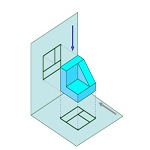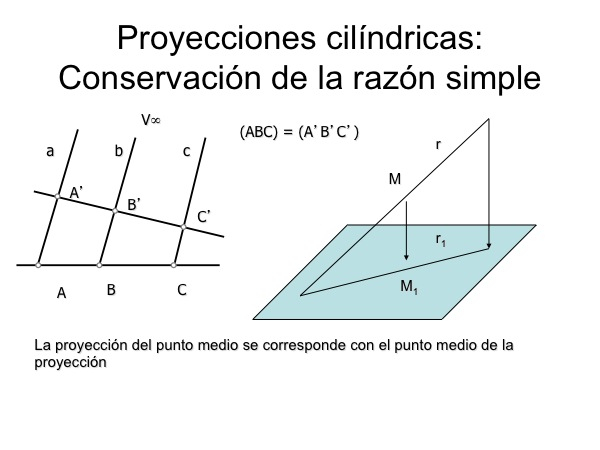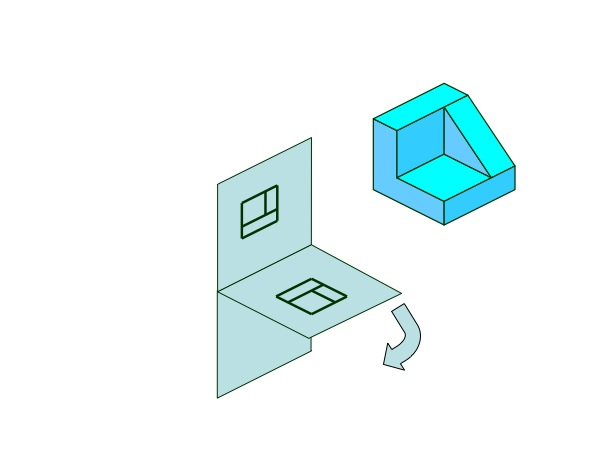# Introduction to technical drawing : NormalizationThe engineering drawing to represent three-dimensional objects on a two-dimensional format (papel) using projection techniques that are studied in the systems of representation, particularly Diédrico System.

Using a cylindrical projection system type (the center of projection is located at infinity) provides interesting advantages from the point of view metric, called simply because it is preserved (derived Thales theorem) in such projections.

The simple reason the three aligned points will express as (ABC), being the ratio of distances from the first point “A” the second “B” and the first “A” the third “C”, namely (ABC)=AB/AC

Due, projecting the midpoint of a segment, it becomes the midpoint of the projection of the segment, allowing to represent objects that are held in these projections relationships distances.Space points take three coordinates (x, and, z) for full determination, so when projecting onto a single plane (two coordinates) lose spatial information. This causes at least two projections on the same plane as necessary to uniquely identify each point.

The model representation we use in technical drawing is based on two projections (the three) on orthogonal planes (perpendicular) together.

The projection on parallel planes does not change the projected shape being a cylindrical system of representation, so that the planes only influence the results based on their spatial orientation, and not its specific position on the object.

After projecting on different projection planes we turn the set on or abate one, so that we get a flat representation.The result of this operation can be on the same plane the two projections of the object. This system of representation is used in engineering to define the objects and their study is accompanied by the standard rules of representation of each individual industry, with a set of general rules for widely.